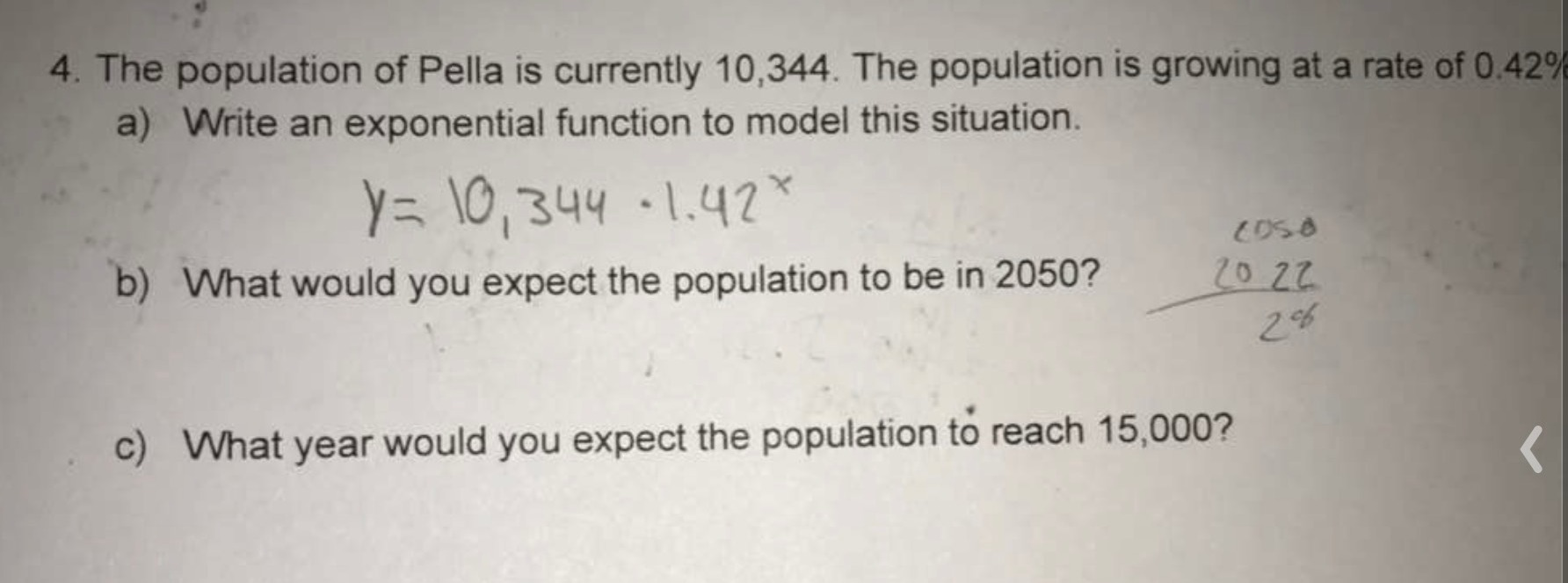### ¿Todavía tienes preguntas de matemáticas?

Pregunte a nuestros tutores expertos
Algebra
Pregunta

4. The population of Pella is currently $$10,344$$ . The population is growing at a rate of $$0.42 \%$$

a) Write an exponential function to model this situation.

$$y = 10,344 \cdot 1.42 ^ { x }$$

b) What would you expect the population to be in $$2050 ? \frac { 20 } { 2 } \%$$

c) What year would you expect the population to reach $$15,000 ?$$a) $$y= 10,344\cdot1.0042^x$$
b) $$10,344\cdot1.0042^{28}= 11632$$
c) $$\log_{1.0042}\frac{15000}{10344}= 89\\89+ 2022= 2111$$